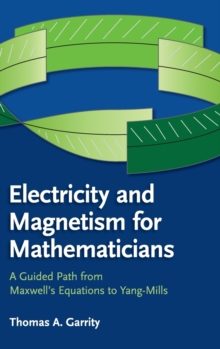Supporting your high street Find out how »
• My AccountElectricity and Magnetism for Mathematicians : A Guided Path from Maxwell's Equations to Yang-Mills Hardback

Description

This text is an introduction to some of the mathematical wonders of Maxwell's equations.

These equations led to the prediction of radio waves, the realization that light is a type of electromagnetic wave, and the discovery of the special theory of relativity.

In fact, almost all current descriptions of the fundamental laws of the universe can be viewed as deep generalizations of Maxwell's equations.

Even more surprising is that these equations and their generalizations have led to some of the most important mathematical discoveries of the past thirty years.

It seems that the mathematics behind Maxwell's equations is endless.

The goal of this book is to explain to mathematicians the underlying physics behind electricity and magnetism and to show their connections to mathematics.

Starting with Maxwell's equations, the reader is led to such topics as the special theory of relativity, differential forms, quantum mechanics, manifolds, tangent bundles, connections, and curvature.

Information

• Format: Hardback
• Pages: 300 pages, Worked examples or Exercises; 2 Plates, color; 81 Line drawings, unspecified
• Publisher: Cambridge University Press
• Publication Date:
• Category: Mathematics
• ISBN: 9781107078208

£67.99

£59.55

on all orders

Pick up orders

from local bookshops